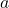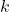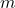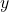>>Interview question: Potato Spies

# Interview question: Potato SpiesIn our initial phone interviews, we like to ask candidates a question based on a real-world scenario, that requires algorithmic or mathematical thinking in order to solve.

Below is just such a test that we wrote but never got round to using. My colleague Kenny and I also presented it to a room full of Oxford Mathematicians in February 2011. Kenny used to work as a teacher, so he grabbed the chalk with relish, while I amused myself throwing new potatoes at the audience whenever they got a question right. That was one careers event they didn’t forget in a hurry!

### Question

Two spies are in the business of communicating with each other through a very primitive communication channel. In fact, they send each other bags of potatoes.

On receiving a bag of potatoes the recipient spy counts how many potatoes he has received and looks up the number in his secret code book in order to decipher the message. He then returns the sack with a different number of potatoes in to reply.

By and large this works very well. No-one is interested in intercepting large bags of potatoes and their conversations go undetected. However, it is noticed that on occasion it would be very useful to be able to send two messages at once. In a brief get-together the spies may be able to agree on a scheme to encode two messages in one bag of potatoes. What could you suggest?

### Discussion

OK. It’s a shaky story at best. What we’re really interested in here is a method of encoding 2 numbers in one. We could of course also send a number of potatoes equal to the higher message and take a bite out of a number of them equal to the smaller message. If the candidate suggests that, we tell them that the potatoes must arrive in pristine condition because the spies sell them to make a living. And smart-arses who point out that you can make invisible ink out of potato juice get binned straight away

There are a number of possible methods outlined below, and the bulk of the interview will be taken up with a comparison of the various methods. The most interesting properties of an encoding method for discussion are the following

• the number of potatoes needed to encode the message
• the ease of and feasibility of encoding and decoding
• the behaviour of the approach with weighted messages (i.e. whether it matters which message comes first)
• extensibility to more than 2 messages.

### Prime decomposition

The Mathematics students among you will probably be tempted to make use of the fact that every number has a unique decomposition into prime factors – so we can encodeandas.

This would work in theory, although in fact it is rather a poor method. Of the methods mentioned here it is the only method which fails to map message pairs 1-1 onto the natural numbers, which means that it’s likely to require more potatoes than the other methods.

To illustrate how bad this method really is, consider that the poor spy wanting to encode 345 and 21 would need approximately 7.497 x 10113 potatoes. Although the more modest 9 and 12 can be encoded in around a quarter of a (US) billion potatoes.

### Concatenation

If we assume that each message is less than a fixed number, then we can encodeandas. Decoding is a simple process of dividing byis the quotient andthe remainder.

If we adopt an appropriate based number system, this process amounts to simple concatenation of the digits ofand the digits of(assuming zero padding where necessary).

For this to be the case, we must adopt a number system with basewhereis a power of.

For example, ifis 1000, the above method is simply a concatenation ofandin decimal with zeros added at the left of each to make them each up to n digits.

345 and 21 become 345021

9 and 12 become 9012

The number of digits required is.

### Interleaving

Concatenation requires us to assume that each message is less than a fixed number. If we cannot make such an assumption, we can still find a solution by “digit manipulation” by interleaving the digits ofand(ababababab…).

For instance:

345 and 21 become 304251

9 and 12 become 192

The number of digits isifandotherwise.

### Plotting

Leaving aside digit manipulation methods we can also view a and b as co-ordinates on a graph. After plottingwe can count outwards from the origin in any of several ways, one obvious one being

(0, 0), (1, 0), (0, 1), (2, 0), (1, 1), (0, 2), (3, 0), (2, 1), (1, 2), (0, 3)…

i.e counting upwards along each of thediagonals in turn.

Using the above method, a and b are encoded asandbecome:

…which is obtained from the formula for triangular numbers plus the remainder left to count on the next diagonal which is thecomponent +1.

The encode process is just counting until you reach the plotted point. The decode process is just counting out the number, plotting the point and reading off the co-ordinates. Of course, for large numbers you’d want to calculate rather than count.

Using this method,

345 and 21 become 67183

9 and 12 become 244

When we presented this at the Oxford Mathematics Institute, we managed to convince ourselves that this method is more efficient than concatenation. However, since we failed to keep a record of the proof, we’ll have to leave that as an exercise to the reader!